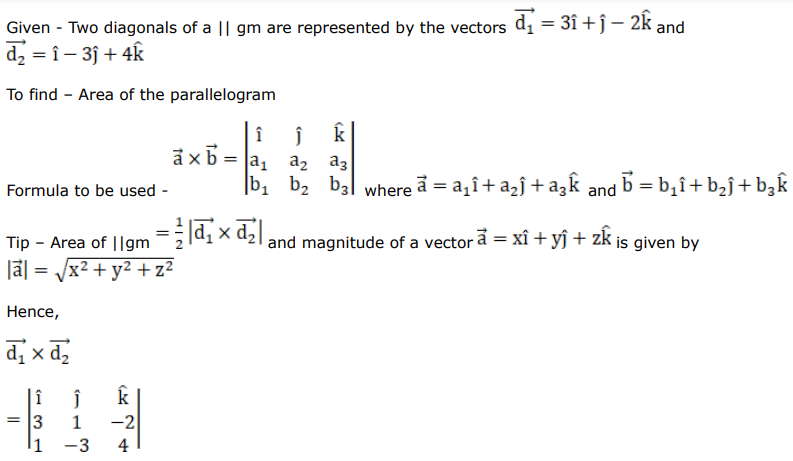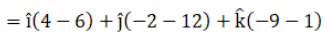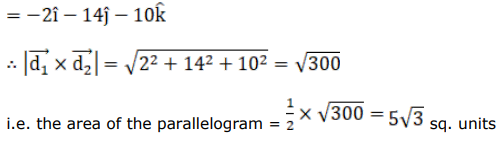# Mark against the correct answer in each of the following:

Question:

Mark $(\sqrt{)}$ against the correct answer in each of the following:

The diagonals of a $\mathrm{II} \mathrm{gm}$ are represented by the vectors $\overrightarrow{\mathrm{d}_{1}}=(3 \hat{\mathrm{i}}+\hat{\mathrm{j}}-2 \hat{\mathrm{k}})$ and $\overrightarrow{\mathrm{d}_{2}}=(\hat{\mathrm{i}}-3 \hat{\mathrm{j}}+4 \hat{\mathrm{k}})$ The area of the II gm is

A. $7 \sqrt{3}$ sq units

B. $5 \sqrt{3}$ sq units

C. $3 \sqrt{5}$ sq units

D. none of these

Solution: